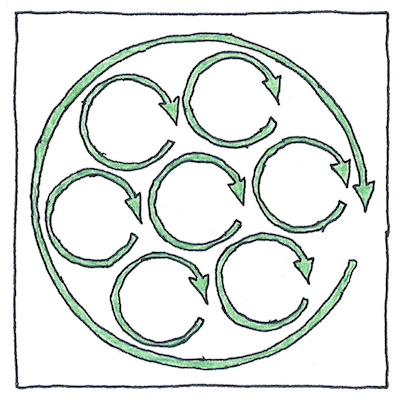# 1864

## The book of science

Tom Sharp

 James Clerk Maxwell electromagnetism

## Maxwell’s equations

• Like little rafts, an electric current
• runs along a river of force vectors
• oriented perpendicular to a surface.
• Bundles of magnetic lines of force
• are like small tubes that carry a magnetic flux
• in arcs above and below a charged surface.
• The work needed to circulate a charge
• around a closed curve in an electric field is related to
• the work needed to circulate a magnetic monopole,
• if one were to exist, in a magnetic field.
• The imputed magnetic monopole
• is a magnet with only one end,
• like an electron, a point in time
• whose negative charge is in our world
• and whose positive charge is in a deformation
• of the field in a minuscule invisible dimension.
• Modern physics can explain everything
• using the properties of fields. Fields, rafts, tubes,
• magnetic monopoles, and numerous invisible dimensions
• are, to those who understand them, like poetry.

## Fields

• The properties of a field can be described
• by a set of numbers associated
• with each point of the field.
• First, mathematicians say a point is a location
• with no extent.
• Second, any extent, however small,
• contains an infinite number of points.
• Third, a field is an extent.
• The relation between reality and a mathematical system
• cannot be determined mathematically.
• The relation rests on the faith
• that each step from the known to the unknown
• might help us recognize something new or useful.
• In particular, it would be useful to explain
• things that our senses
• cannot determine—how gravity
• acts at a distance, why light
• travels at a certain speed, and whether the universe
• will end in fire or in ice.

## James Clerk Maxwell

• James Clerk, named Maxwell after his mother died,
• studied at Edinburg, Cambridge, and Trinity,
• earned honors, prizes, and a mathematics degree at 23;
• taught at Marischal College and married the daughter of the principal,
• planned the famous Cavendish laboratory
• and became the first Cavendish professor at Cambridge,
• was the first to use probability and statistics
• to explain the behavior of gases,
• studied color blindness and color vision,
• resulting in the first color photograph,
• extended Michael Faraday’s theories of electromagnetism
• suggesting that “light consists in the transverse undulations
• of the same medium which is the cause
• of electric and magnetic phenomenon.”
• Nine years after he died in 1879 at 48,
• according to his theory, Heinrich Hertz
• demonstrated that an electric disturbance
• is transmitted through space in waves,
• and, nearly one-hundred years after he died,
• NASA’s Voyager space probe
• confirmed Maxwell’s theory that the rings of Saturn
• are composed of many small particles.

James Clerk Maxwell was the first to realize how electricity, magnetism, and light are manifestations of the same physical forces—electromagnetic radiation—described as photons or waves with different energies, wavelength, and frequencies. As Einstein wrote, Maxwell realized “that electromagnetic fields spread in the form of polarised waves, and at the speed of light!” Maxwell wrote that “light consists in the transverse undulations of the same medium which is the cause of electric and magnetic phenomena.”

Electric charges and currents create electric and magnetic fields (physical lines of force) that can be described mathematically. Maxwell’s equations describe and improve work by Carl Friedrich Gauss, Micael Faraday, and André-Marie Ampère, that is, Gauss’s law, Gauss’s law for magnetism, Faraday’s law of induction, and Ampère’s circuital law.

See also in The book of science:

Readings on wikipedia: